Documentation

pdeplot

Plot solution or mesh for 2-D problem

Description

example

pdeplot(model,'XYData',results.NodalSolution) plots the solution of a model at nodal locations as a colored surface plot using the default 'jet' colormap.

example

pdeplot(model,'XYData',results.Temperature,'ColorMap','hot') plots the temperature at nodal locations for a 2-D thermal analysis model. This syntax creates a colored surface plot using the 'hot' colormap.

example

pdeplot(model,'XYData',results.VonMisesStress,'Deformation',results.Displacement) plots the von Mises stress and shows the deformed shape for a 2-D structural analysis model.

example

pdeplot(model,'XYData',results.ModeShapes.ux) plots the x-component of the modal displacement for a 2-D structural modal analysis model.

example

pdeplot(model) plots the mesh specified in model.

example

pdeplot(mesh) plots the mesh defined as a Mesh property of a 2-D model object of type PDEModel.

example

pdeplot(nodes,elements) plots the mesh defined by its nodes and elements.

example

pdeplot(p,e,t) plots the mesh described by p,e, and t.

example

pdeplot(___,Name,Value) plots the mesh, the data at the nodal locations, or both the mesh and the data, depending on the Name,Value pair arguments. Use any arguments from the previous syntaxes.

Specify at least one of the FlowData (vector field plot), XYData (colored surface plot), or ZData (3-D height plot) name-value pairs. Otherwise, pdeplot plots the mesh with no data. You can combine any number of plot types.

• For a thermal model, you can plot temperature or gradient of temperature.

• For a structural model, you can plot displacement, stress, strain, and von Mises stress. In addition, you can show the deformed shape and specify the scaling factor for the deformation plot.

example

h = pdeplot(___) returns a handle to a plot, using any of the previous syntaxes.

Examples

collapse all

Create a PDE model. Include the geometry of the built-in function lshapeg. Mesh the geometry and plot it.

model = createpde;
geometryFromEdges(model,@lshapeg);
mesh = generateMesh(model);
pdeplot(model)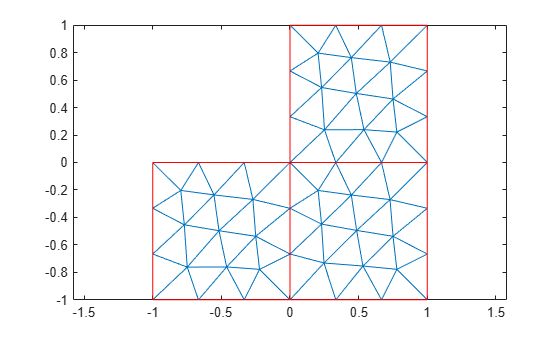Alternatively, you can plot a mesh by using mesh as an input argument.

pdeplot(mesh)Another approach is to use the nodes and elements of the mesh as input arguments for pdeplot.

pdeplot(mesh.Nodes,mesh.Elements)Display the node labels. Use xlim and ylim to zoom in on particular nodes.

pdeplot(model,'NodeLabels','on')
xlim([-0.2,0.2])
ylim([-0.2,0.2])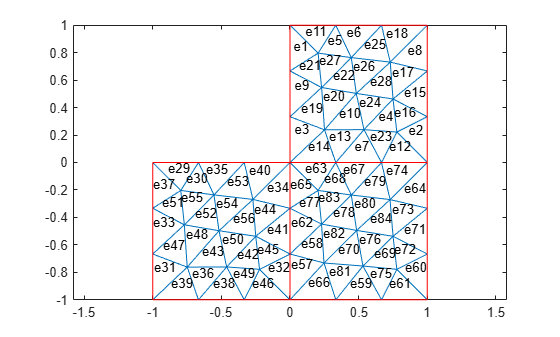Display the element labels.

pdeplot(model,'ElementLabels','on')
xlim([-0.2,0.2])
ylim([-0.2,0.2])Create colored 2-D and 3-D plots of a solution to a PDE model.

Create a PDE model. Include the geometry of the built-in function lshapeg. Mesh the geometry.

model = createpde;
geometryFromEdges(model,@lshapeg);
generateMesh(model);

Set the zero Dirichlet boundary conditions on all edges.

applyBoundaryCondition(model,'dirichlet','Edge',1:model.Geometry.NumEdges,'u',0);

Specify the coefficients and solve the PDE.

specifyCoefficients(model,'m',0, ...
'd',0, ...
'c',1, ...
'a',0, ...
'f',1);
results = solvepde(model)
results =
StationaryResults with properties:

NodalSolution: [1177x1 double]
Mesh: [1x1 FEMesh]

Access the solution at the nodal locations.

u = results.NodalSolution;

Plot the 2-D solution.

pdeplot(model,'XYData',u)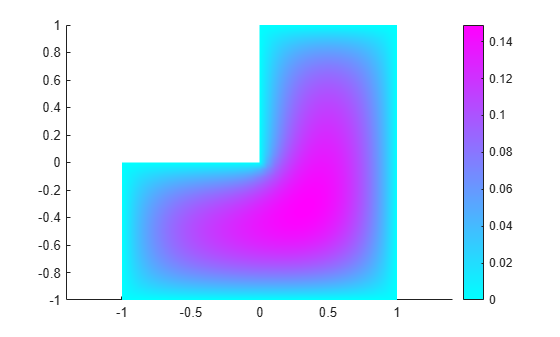Plot the 3-D solution.

pdeplot(model,'XYData',u,'ZData',u)Plot the gradient of a PDE solution as a quiver plot.

Create a PDE model. Include the geometry of the built-in function lshapeg. Mesh the geometry.

model = createpde;
geometryFromEdges(model,@lshapeg);
generateMesh(model);

Set the zero Dirichlet boundary conditions on all edges.

applyBoundaryCondition(model,'dirichlet','Edge',1:model.Geometry.NumEdges,'u',0);

Specify coefficients and solve the PDE.

specifyCoefficients(model,'m',0, ...
'd',0, ...
'c',1, ...
'a',0, ...
'f',1);
results = solvepde(model)
results =
StationaryResults with properties:

NodalSolution: [1177x1 double]
Mesh: [1x1 FEMesh]

Access the gradient of the solution at the nodal locations.

Plot the gradient as a quiver plot.

pdeplot(model,'FlowData',[ux,uy])Plot the solution of a 2-D PDE in 3-D with the 'jet' coloring and a mesh, and include a quiver plot. Get handles to the axes objects.

Create a PDE model. Include the geometry of the built-in function lshapeg. Mesh the geometry.

model = createpde;
geometryFromEdges(model,@lshapeg);
generateMesh(model);

Set zero Dirichlet boundary conditions on all edges.

applyBoundaryCondition(model,'dirichlet','Edge',1:model.Geometry.NumEdges,'u',0);

Specify coefficients and solve the PDE.

specifyCoefficients(model,'m',0, ...
'd',0, ...
'c',1, ...
'a',0, ...
'f',1);
results = solvepde(model)
results =
StationaryResults with properties:

NodalSolution: [1177x1 double]
Mesh: [1x1 FEMesh]

Access the solution and its gradient at the nodal locations.

u = results.NodalSolution;

Plot the solution in 3-D with the 'jet' coloring and a mesh, and include the gradient as a quiver plot.

h = pdeplot(model,'XYData',u,'ZData',u, ...
'FaceAlpha',0.5, ...
'FlowData',[ux,uy], ...
'ColorMap','jet', ...
'Mesh','on')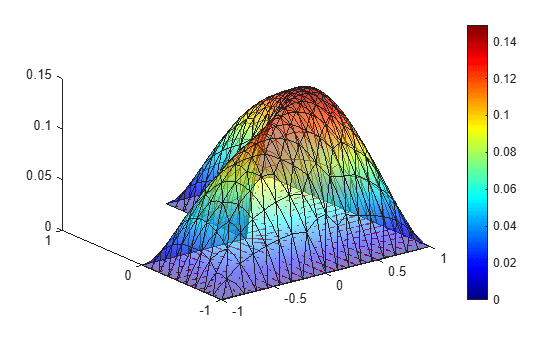h =
3x1 graphics array:

Patch
Quiver
ColorBar

Solve a 2-D transient thermal problem.

Create a transient thermal model for this problem.

thermalmodel = createpde('thermal','transient');

Create the geometry and include it in the model.

SQ1 = [3; 4; 0; 3; 3; 0; 0; 0; 3; 3];
D1 = [2; 4; 0.5; 1.5; 2.5; 1.5; 1.5; 0.5; 1.5; 2.5];
gd = [SQ1 D1];
sf = 'SQ1+D1';
ns = char('SQ1','D1');
ns = ns';
dl = decsg(gd,sf,ns);
geometryFromEdges(thermalmodel,dl);
pdegplot(thermalmodel,'EdgeLabels','on','FaceLabels','on')
xlim([-1.5 4.5])
ylim([-0.5 3.5])
axis equal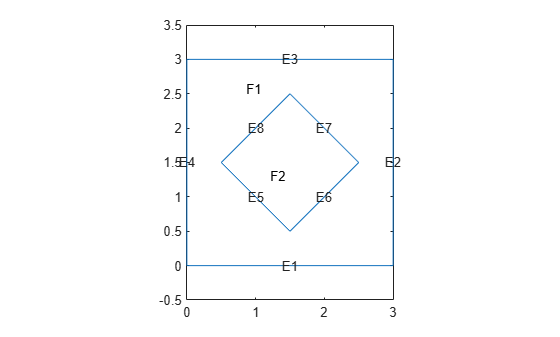For the square region, assign these thermal properties:

• Thermal conductivity is $10\phantom{\rule{0.16666666666666666em}{0ex}}W/\left({m}^{\circ }C\right)$

• Mass density is $2\phantom{\rule{0.16666666666666666em}{0ex}}kg/{m}^{3}$

• Specific heat is $0.1\phantom{\rule{0.16666666666666666em}{0ex}}J/\left(k{g}^{\circ }C\right)$

thermalProperties(thermalmodel,'ThermalConductivity',10, ...
'MassDensity',2, ...
'SpecificHeat',0.1, ...
'Face',1);

For the diamond region, assign these thermal properties:

• Thermal conductivity is $2\phantom{\rule{0.16666666666666666em}{0ex}}W/\left({m}^{\circ }C\right)$

• Mass density is $1\phantom{\rule{0.16666666666666666em}{0ex}}kg/{m}^{3}$

• Specific heat is $0.1\phantom{\rule{0.16666666666666666em}{0ex}}J/\left(k{g}^{\circ }C\right)$

thermalProperties(thermalmodel,'ThermalConductivity',2, ...
'MassDensity',1, ...
'SpecificHeat',0.1, ...
'Face',2);

Assume that the diamond-shaped region is a heat source with a density of $4\phantom{\rule{0.16666666666666666em}{0ex}}W/{m}^{3}$.

internalHeatSource(thermalmodel,4,'Face',2);

Apply a constant temperature of $0{\phantom{\rule{0.16666666666666666em}{0ex}}}^{\circ }C$ to the sides of the square plate.

thermalBC(thermalmodel,'Temperature',0,'Edge',[1 2 7 8]);

Set the initial temperature to $0{\phantom{\rule{0.16666666666666666em}{0ex}}}^{\circ }C$.

thermalIC(thermalmodel,0);

Mesh the geometry.

generateMesh(thermalmodel);

The dynamics for this problem are very fast. The temperature reaches a steady state in about 0.1 seconds. To capture the interesting part of the dynamics, set the solution time to logspace(-2,-1,10). This command returns 10 logarithmically spaced solution times between 0.01 and 0.1.

tlist = logspace(-2,-1,10);

Solve the equation.

thermalresults = solve(thermalmodel,tlist)
thermalresults =
TransientThermalResults with properties:

Temperature: [1481x10 double]
SolutionTimes: [1x10 double]
Mesh: [1x1 FEMesh]

Plot the solution with isothermal lines by using a contour plot.

T = thermalresults.Temperature;
pdeplot(thermalmodel,'XYData',T(:,10),'Contour','on','ColorMap','hot')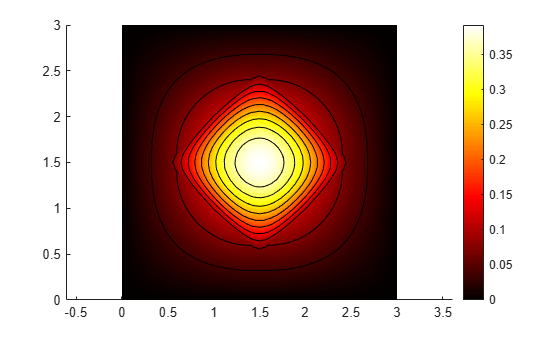Create a structural analysis model for a static plane-strain problem.

structuralmodel = createpde('structural','static-planestrain');

Create the geometry and include it in the model. Plot the geometry.

geometryFromEdges(structuralmodel,@squareg);
pdegplot(structuralmodel,'EdgeLabels','on')
axis equalSpecify the Young's modulus and Poisson's ratio.

structuralProperties(structuralmodel,'PoissonsRatio',0.3, ...
'YoungsModulus',210E3);

Specify the x-component of the enforced displacement for edge 1.

structuralBC(structuralmodel,'XDisplacement',0.001,'Edge',1);

Specify that edge 3 is a fixed boundary.

structuralBC(structuralmodel,'Constraint','fixed','Edge',3);

Generate a mesh and solve the problem.

generateMesh(structuralmodel);
structuralresults = solve(structuralmodel);

Plot the deformed shape using the default scale factor. By default, pdeplot internally determines the scale factor based on the dimensions of the geometry and the magnitude of deformation.

pdeplot(structuralmodel,'XYData',structuralresults.VonMisesStress, ...
'Deformation',structuralresults.Displacement, ...
'ColorMap','jet')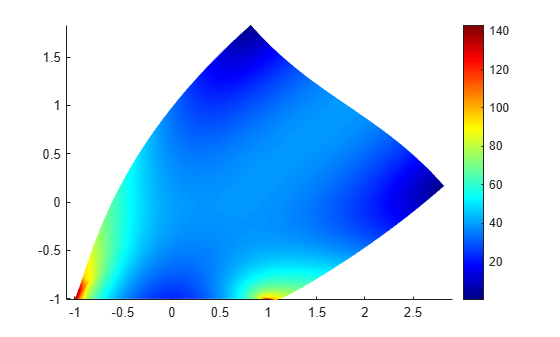Plot the deformed shape with the scale factor 500.

pdeplot(structuralmodel,'XYData',structuralresults.VonMisesStress, ...
'Deformation',structuralresults.Displacement, ...
'DeformationScaleFactor',500,...
'ColorMap','jet')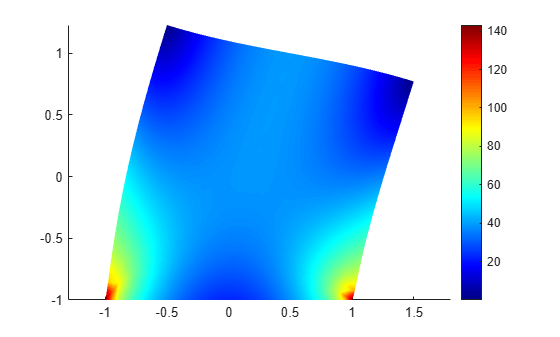Plot the deformed shape without scaling.

pdeplot(structuralmodel,'XYData',structuralresults.VonMisesStress, ...
'ColorMap','jet')Find the fundamental (lowest) mode of a 2-D cantilevered beam, assuming prevalence of the plane-stress condition.

Specify the following geometric and structural properties of the beam, along with a unit plane-stress thickness.

length = 5;
height = 0.1;
E = 3E7;
nu = 0.3;
rho = 0.3/386;

Create a model plane-stress model, assign a geometry, and generate a mesh.

structuralmodel = createpde('structural','modal-planestress');
gdm = [3;4;0;length;length;0;0;0;height;height];
g = decsg(gdm,'S1',('S1')');
geometryFromEdges(structuralmodel,g);

Define a maximum element size (five elements through the beam thickness).

hmax = height/5;
msh=generateMesh(structuralmodel,'Hmax',hmax);

Specify the structural properties and boundary constraints.

structuralProperties(structuralmodel,'YoungsModulus',E, ...
'MassDensity',rho, ...
'PoissonsRatio',nu);
structuralBC(structuralmodel,'Edge',4,'Constraint','fixed');

Compute the analytical fundamental frequency (Hz) using the beam theory.

I = height^3/12;
analyticalOmega1 = 3.516*sqrt(E*I/(length^4*(rho*height)))/(2*pi)
analyticalOmega1 = 126.9498

Specify a frequency range that includes an analytically computed frequency and solve the model.

modalresults = solve(structuralmodel,'FrequencyRange',[0,1e6])
modalresults =
ModalStructuralResults with properties:

NaturalFrequencies: [32x1 double]
ModeShapes: [1x1 struct]
Mesh: [1x1 FEMesh]

The solver finds natural frequencies and modal displacement values at nodal locations. To access these values, use modalresults.NaturalFrequencies and modalresults.ModeShapes.

modalresults.NaturalFrequencies/(2*pi)
ans = 32×1
105 ×

0.0013
0.0079
0.0222
0.0433
0.0711
0.0983
0.1055
0.1462
0.1930
0.2455
⋮

modalresults.ModeShapes
ans = struct with fields:
ux: [6511x32 double]
uy: [6511x32 double]

Plot the y-component of the solution for the fundamental frequency.

pdeplot(structuralmodel,'XYData',modalresults.ModeShapes.uy(:,1))
title(['First Mode with Frequency ', ...
num2str(modalresults.NaturalFrequencies(1)/(2*pi)),' Hz'])
axis equal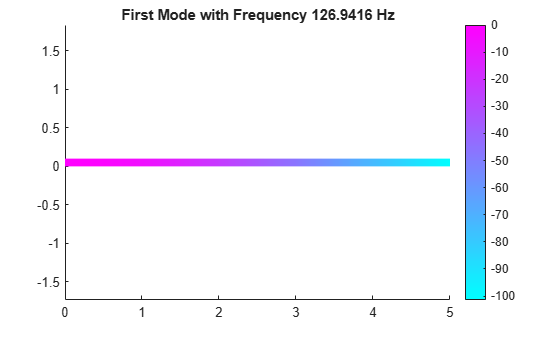Plot the p,e,t mesh. Display the solution using 2-D and 3-D colored plots.

Create the geometry, mesh, boundary conditions, PDE coefficients, and solution.

[p,e,t] = initmesh('lshapeg');
u = assempde('lshapeb',p,e,t,1,0,1);

Plot the mesh.

pdeplot(p,e,t)Plot the solution as a 2-D colored plot.

pdeplot(p,e,t,'XYData',u)Plot the solution as a 3-D colored plot.

pdeplot(p,e,t,'XYData',u,'ZData',u)Input Arguments

collapse all

Model object, specified as a PDEModel object, ThermalModel object, or StructuralModel object.

Example: model = createpde(1)

Example: thermalmodel = createpde('thermal','steadystate')

Example: structuralmodel = createpde('structural','static-solid')

Mesh object, specified as the Mesh property of a PDEModel object or as the output of generateMesh.

Example: model.Mesh

Nodal coordinates, specified as a 2-by-NumNodes matrix. NumNodes is the number of nodes.

Element connectivity matrix in terms of the node IDs, specified as a 3-by-NumElements or 6-by-NumElements matrix. Linear meshes contain only corner nodes. For linear meshes, the connectivity matrix has three nodes per 2-D element. Quadratic meshes contain corner nodes and nodes in the middle of each edge of an element. For quadratic meshes, the connectivity matrix has six nodes per 2-D element.Mesh points, specified as a 2-by-Np matrix of points, where Np is the number of points in the mesh. For a description of the (p,e,t) matrices, see Mesh Data.

Typically, you use the p, e, and t data exported from the PDE Modeler app, or generated by initmesh or refinemesh.

Example: [p,e,t] = initmesh(gd)

Data Types: double

Mesh edges, specified as a 7-by-Ne matrix of edges, where Ne is the number of edges in the mesh. For a description of the (p,e,t) matrices, see Mesh Data.

Typically, you use the p, e, and t data exported from the PDE Modeler app, or generated by initmesh or refinemesh.

Example: [p,e,t] = initmesh(gd)

Data Types: double

Mesh triangles, specified as a 4-by-Nt matrix of triangles, where Nt is the number of triangles in the mesh. For a description of the (p,e,t) matrices, see Mesh Data.

Typically, you use the p, e, and t data exported from the PDE Modeler app, or generated by initmesh or refinemesh.

Example: [p,e,t] = initmesh(gd)

Data Types: double

Name-Value Pair Arguments

Specify optional comma-separated pairs of Name,Value arguments. Name is the argument name and Value is the corresponding value. Name must appear inside quotes. You can specify several name and value pair arguments in any order as Name1,Value1,...,NameN,ValueN.

Example: pdeplot(model,'XYData',u,'ZData',u)

When you use a PDEModel object, pdeplot(model,'XYData',u,'ZData',u) sets surface plot coloring to the solution u, and sets the heights for a 3-D plot to u. Here u is a NodalSolution property of the PDE results returned by solvepde or solvepdeeig.

When you use a [p,e,t] representation, pdeplot(p,e,t,'XYData',u,'ZData',u) sets surface plot coloring to the solution u and sets the heights for a 3-D plot to the solution u. Here u is a solution returned by a legacy solver, such as assempde.

Tip

Specify at least one of the FlowData (vector field plot), XYData (colored surface plot), or ZData (3-D height plot) name-value pairs. Otherwise, pdeplot plots the mesh with no data.

Data Plots

collapse all

Colored surface plot data, specified as the comma-separated pair consisting of 'XYData' and a vector. If you use a [p,e,t] representation, specify data for points in a vector of length size(p,2), or specify data for triangles in a vector of length size(t,2).

• Typically, you set XYData to the solution u. The pdeplot function uses XYData for coloring both 2-D and 3-D plots.

• pdeplot uses the colormap specified in the ColorMap name-value pair, using the style specified in the XYStyle name-value pair.

• When the Contour name-value pair is 'on', pdeplot also plots level curves of XYData.

• pdeplot plots the real part of complex data.

To plot the kth component of a solution to a PDE system, extract the relevant part of the solution. For example, when using a PDEModel object, specify:

results = solvepde(model);
u = results.NodalSolution; % each column of u has one component of u
pdeplot(model,'XYData',u(:,k)) % data for column k

When using a [p,e,t] representation, specify:

np = size(p,2); % number of node points
uk = reshape(u,np,[]); % each uk column has one component of u
pdeplot(p,e,t,'XYData',uk(:,k)) % data for column k

Example: 'XYData',u

Data Types: double

Coloring choice, specified as the comma-separated pair consisting of 'XYStyle' and 'interp', 'off', or 'flat'.

• 'off' — No shading, only mesh is displayed.

• 'flat' — Each triangle in the mesh has a uniform color.

• 'interp' — Plot coloring is smoothly interpolated.

The coloring choice relates to the XYData name-value pair.

Example: 'XYStyle','flat'

Data Types: char | string

Data for the 3-D plot heights, specified as the comma-separated pair consisting of 'ZData' and a matrix. If you use a [p,e,t] representation, provide data for points in a vector of length size(p,2) or data for triangles in a vector of length size(t,2).

• Typically, you set ZData to u, the solution. The XYData name-value pair sets the coloring of the 3-D plot.

• The ZStyle name-value pair specifies whether the plot is continuous or discontinuous.

• pdeplot plots the real part of complex data.

To plot the kth component of a solution to a PDE system, extract the relevant part of the solution. For example, when using a PDEModel object, specify:

results = solvepde(model);
u = results.NodalSolution; % each column of u has one component of u
pdeplot(model,'XYData',u(:,k),'ZData',u(:,k)) % data for column k

When using a [p,e,t] representation, specify:

np = size(p,2); % number of node points
uk = reshape(u,np,[]); % each uk column has one component of u
pdeplot(p,e,t,'XYData',uk(:,k),'ZData',uk(:,k)) % data for column k

Example: 'ZData',u

Data Types: double

3-D plot style, specified as the comma-separated pair consisting of 'ZStyle' and one of these values:

• 'off' — No 3-D plot.

• 'discontinuous' — Each triangle in the mesh has a uniform height in a 3-D plot.

• 'continuous' — 3-D surface plot is continuous.

If you use ZStyle without specifying the ZData name-value pair, then pdeplot ignores ZStyle.

Example: 'ZStyle','discontinuous'

Data Types: char | string

Data for the quiver plot, specified as the comma-separated pair consisting of 'FlowData' and an M-by-2 matrix, where M is the number of mesh nodes. FlowData contains the x and y values of the field at the mesh points.

When you use a PDEModel object, set FlowData as follows:

results = solvepde(model);

When you use a [p,e,t] representation, set FlowData as follows:

When you use ZData to represent a 2-D PDE solution as a 3-D plot and you also include a quiver plot, the quiver plot appears in the z = 0 plane.

pdeplot plots the real part of complex data.

Example: 'FlowData',[ux uy]

Data Types: double

Indicator to show the quiver plot, specified as the comma-separated pair consisting of 'FlowStyle' and 'arrow' or 'off'. Here, 'arrow' displays the quiver plot specified by the FlowData name-value pair.

Example: 'FlowStyle','off'

Data Types: char | string

Indicator to convert the mesh data to x-y grid before plotting, specified as the comma-separated pair consisting of 'XYGrid' and 'off' or 'on'.

Note

This conversion can change the geometry and lessen the quality of the plot.

By default, the grid has about sqrt(size(t,2)) elements in each direction.

Example: 'XYGrid','on'

Data Types: char | string

Customized x-y grid, specified as the comma-separated pair consisting of 'GridParam' and a matrix [tn;a2;a3]. For example:

[~,tn,a2,a3] = tri2grid(p,t,u,x,y);
pdeplot(p,e,t,'XYGrid','on','GridParam',[tn;a2;a3],'XYData',u)

For details on the grid data and its x and y arguments, see tri2grid. The tri2grid function does not work with PDEModel objects.

Example: 'GridParam',[tn;a2;a3]

Data Types: double

Mesh Plots

collapse all

Node labels, specified as the comma-separated pair consisting of 'NodeLabels' and 'off' or 'on'.

pdeplot ignores NodeLabels when you use it with ZData.

Example: 'NodeLabels','on'

Data Types: char | string

Element labels, specified as the comma-separated pair consisting of 'ElementLabels' and 'off' or 'on'.

pdeplot ignores ElementLabels when you use it with ZData.

Example: 'ElementLabels','on'

Data Types: char | string

Structural Analysis Plots

collapse all

Data for plotting the deformed shape for a structural analysis model, specified as the comma-separated pair consisting of 'Deformation' and the Displacement property of the StaticStructuralResults object. This property is a structure array with the fields containing displacement components at the nodal locations.

Example: 'Deformation',structuralresults.Displacement

Data Types: struct

Scaling factor for plotting the deformed shape, specified as the comma-separated pair consisting of 'DeformationScaleFactor' and a real number. Use this argument with the Deformation name-value pair. The default value is defined internally, based on the dimensions of the geometry and the magnitude of the deformation.

Example: 'DeformationScaleFactor',100

Data Types: double

Annotations and Appearance

collapse all

Indicator to include a color bar, specified as the comma-separated pair consisting of 'ColorBar' and 'on' or 'off'. Specify 'on' to display a bar giving the numeric values of colors in the plot. For details, see colorbar. The pdeplot function uses the colormap specified in the ColorMap name-value pair.

Example: 'ColorBar','off'

Data Types: char | string

Colormap, specified as the comma-separated pair consisting of 'ColorMap' and a value representing a built-in colormap, or a colormap matrix. For details, see colormap.

ColorMap must be used with the XYData name-value pair.

Example: 'ColorMap','jet'

Data Types: double | char | string

Indicator to show the mesh, specified as the comma-separated pair consisting of 'Mesh' and 'on' or 'off'. Specify 'on' to show the mesh in the plot.

Example: 'Mesh','on'

Data Types: char | string

Title of plot, specified as the comma-separated pair consisting of 'Title' and a character vector.

Example: 'Title','Solution Plot'

Data Types: char | string

Surface transparency for 3-D geometry, specified as the comma-separated pair consisting of 'FaceAlpha' and a real number from 0 through 1. The default value 1 indicates no transparency. The value 0 indicates complete transparency.

Example: 'FaceAlpha',0.5

Data Types: double

Indicator to plot level curves, specified as the comma-separated pair consisting of 'Contour' and 'off' or 'on'. Specify 'on' to plot level curves for the XYData data. Specify the levels with the Levels name-value pair.

Example: 'Contour','on'

Data Types: char | string

Levels for contour plot, specified as the comma-separated pair consisting of 'Levels' and a positive integer or a vector of level values.

• Positive integer — Plot Levels as equally spaced contours.

• Vector — Plot contours at the values in Levels.

To obtain a contour plot, set the Contour name-value pair to 'on'.

Example: 'Levels',16

Data Types: double

Output Arguments

collapse all

Handles to graphics objects, returned as a vector.

collapse all

Quiver Plot

A quiver plot is a plot of a vector field. It is also called a flow plot.

Arrows show the direction of the field, with the lengths of the arrows showing the relative sizes of the field strength. For details on quiver plots, see quiver.

Introduced before R2006a

Partial Differential Equation Toolbox DocumentationGet trial now